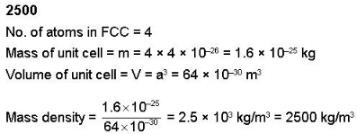Courses

# IIT JAM Physics MCQ Test 18

## 60 Questions MCQ Test Mock Test Series for IIT JAM Physics | IIT JAM Physics MCQ Test 18

Description
This mock test of IIT JAM Physics MCQ Test 18 for Physics helps you for every Physics entrance exam. This contains 60 Multiple Choice Questions for Physics IIT JAM Physics MCQ Test 18 (mcq) to study with solutions a complete question bank. The solved questions answers in this IIT JAM Physics MCQ Test 18 quiz give you a good mix of easy questions and tough questions. Physics students definitely take this IIT JAM Physics MCQ Test 18 exercise for a better result in the exam. You can find other IIT JAM Physics MCQ Test 18 extra questions, long questions & short questions for Physics on EduRev as well by searching above.
QUESTION: 1

### In a common emitter amplifier, the unbypassed emitter resistance provide:-

Solution:

There are two circuits with emitter resistance unbypassed. In CE amplifier the output is taken from collector. Here, the feedback is current series.

QUESTION: 2

### In the following given circuit, calculate the value of "Vce" if the transistor is working in cut off:-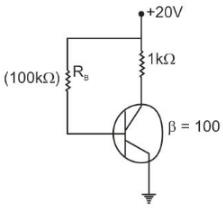Solution:

As the transistor is working in cut off region.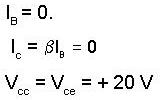QUESTION: 3

### A change in the value of the emitter resistance (Re), in a differential amplifier:-

Solution:

(Emitter resistance only affects the common mode output & thus the common mode gain)

QUESTION: 4

The ripple factor of a power supply is given by (symbols have the usual meaning)

Solution:

The magnitude of the ripple is given by the ratio of the root mean square value of the ac component to the mean value of the total & is usually expressed as a percentage. This is known as ripple factor.

QUESTION: 5

A diode has a reverse saturation current Is = 10-10 A & non-ideality factor η = 2. If diode voltage is 0.9 V then diode current is:-

Solution: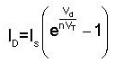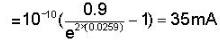QUESTION: 6

An op-amp has a slow-rate 5V/μs. The largest sinwave output voltage possible at a frequency of 1MHz is:-

Solution: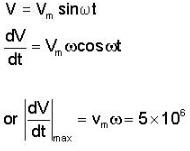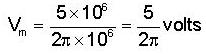QUESTION: 7

The form factor (in case of resistive load) for a full wave rectifier is:-

Solution:

Form factor(F) =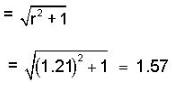QUESTION: 8

The static characteristic of an adequately forward biased p-n junction is a straight line, if the plot is of:

Solution:

Static characteristic of a forward biased p-n junction :-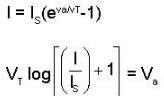Thus straight line is : log I v/s V

QUESTION: 9

The ideal characteristic of a stabilizer is:-

Solution:

Voltage stabilizer is a zener diode or other device that suppresses variation in a DC voltage. It is often used in place of capacitor, across a cathode biasing resistor.

QUESTION: 10

Silicon diode is less suited for low voltage rectifier operation, because:-

Solution:

Solicon diode is less suited for low voltage rectifier operation because its break down voltage is high

QUESTION: 11

The breakdown voltage of a transistor with its base open is BVCEO & that with emitter open is BVCBO, then

Solution:

Become the emitter is most heavily doped region in the transistor. It is about 40 to 50% BVCBO

QUESTION: 12

In a bipolar transistor at room temperature, if the emitter current is doubled the voltage across its base emitter junction will

Solution:

In a BJT emitter current is doubled and one has to find the base emitter voltage, so we consider the p – n junction formed by emitter and base. The junction current is given by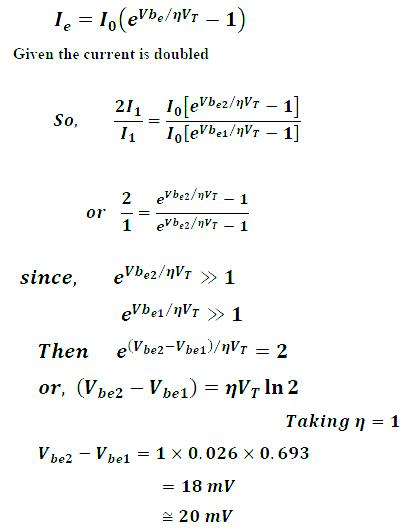QUESTION: 13

What is the current through 100Ω resistance is the circuit shown below?Solution:

The current will not pass through Z2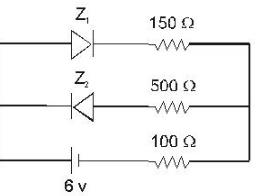Hence, 6 = I(150+100)
6 = 250 I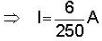= 0.024 A

QUESTION: 14

Which of the following statements if not true regarding the magnitude of barrier potential of a p-n junction.

Solution:

When a p-type semiconductor having holes as majority carrier is clamped with a n-type semiconductor having electron as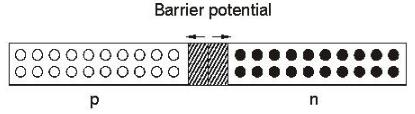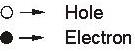Majority carrier some electrons from n side goes to p side and some holes goes to p side and some holes goes to n side, thus created a potential barrier, which
(i) Depends on temperature
(ii) Depends on Fermi level
(iii) Forbidden energy gap Eg
(iv) Majority carriers.

QUESTION: 15

If in a p-n junction diode, a square input signal of 6V is applied see figure be-low, what is the value of the output signal across 'RL'?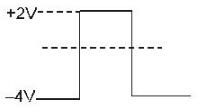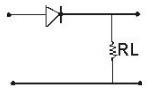Solution: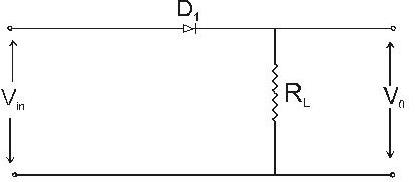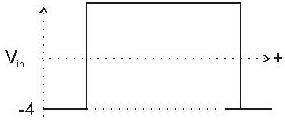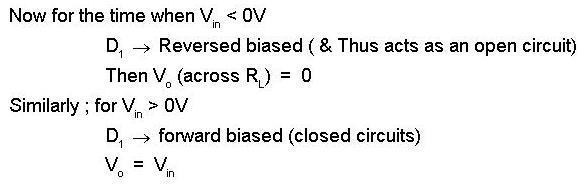Thus output curve will be:-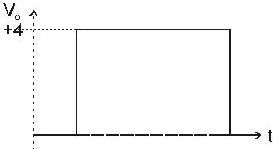QUESTION: 16

The circuit shown below has two oppositely connected ideal diodes in parallel. What is the current flowing in the circuit?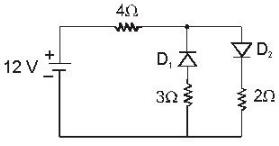Solution: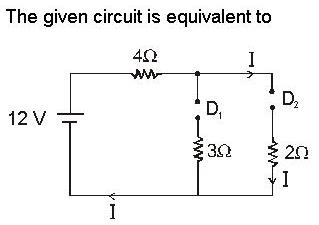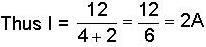QUESTION: 17

The input signal given to a CE amplifier having a voltage gain of 150 is V1 = 2cos(15t+100). The corresponding output signal is:

Solution:

The voltage gain is defined as:
12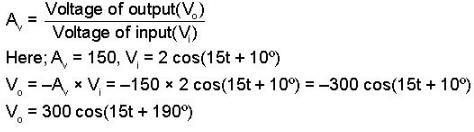QUESTION: 18

In the following circuit, the voltage across & the current through the 2kΩ resistance are: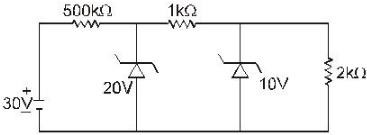Solution:

Zener diode acts a DC voltage stabilizer. Hence the voltage developed across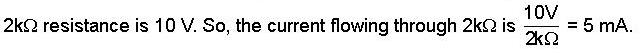QUESTION: 19

In the following circuit Tr1 & Tr2 are identical transistors having VBE = 0.7V. The current passing through the transistor Tr2 is :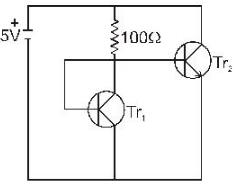Solution:

Applying KVL, we get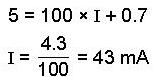QUESTION: 20

A bipolar junction transistors with one junction forward biased & either the collector or emitter open, operated in the :

Solution:

When one junction is forward biased either collector or emitter open, operates in pinch-off region.

QUESTION: 21

A  silicon diode is in series with a 1.0 kΩ resistor and a 5V battery. If the anode is connected to the positive battery terminal, the cathode voltage with respect to the negative battery terminal is:

Solution:

The cathode voltage with respect to the negative battery terminal is VBias-VF for silicon VF = 0.7 V, VBias = 5V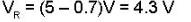QUESTION: 22

Which one of the following statement is true for a semiconductor p-n junction with no external bias?

Solution:

In case of SC p-n junction with no external bias: the structure looks like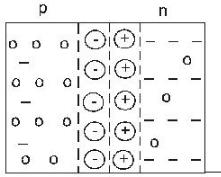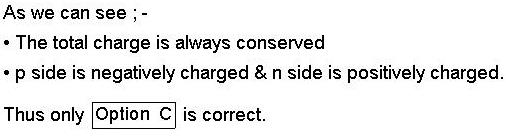QUESTION: 23

An intrinsic semiconducting sample has an energy gap of 0.38eV, calculate the probability at 1000C that the lowest level in the conduction band will be occupied.

Solution: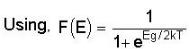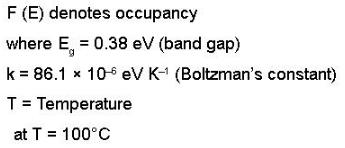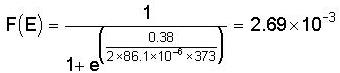QUESTION: 24

Which of the following output curves correctly  represent the output of the given clamper circuit:-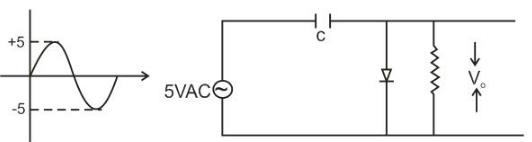Solution: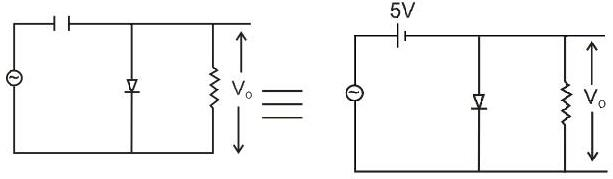The correct answer is option B.

QUESTION: 25

Which relation is correct for intrinsic semiconductor?
(where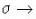conducting of material μn and μp is the electron mobility and hole mobility respectively.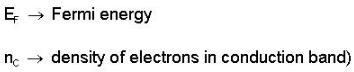Solution:

The correct relation is given by,

σ=z exp(-Eg/2KBT)e(μnp)

and, nc=2(2πm,eKBT/h2)3/2 exp[(Ef-Ec)/KBT]

The correct answer is option C.

QUESTION: 26

If the transistor of an amplifier is changed & the gain is increased by two times, which of the following Parameters will have the higher increase in its value :-

Solution: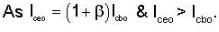QUESTION: 27

Mobility of free electrons depends on temperature variation in case of a semiconductor as:-

Solution: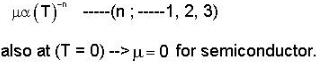QUESTION: 28

The graph of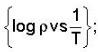where P → Resistinity & 'T' is the temperature :-

Solution: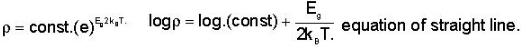QUESTION: 29

In a germanium semiconductor material used in transistors, mobility of electrons is 3900 cm2N. How much velocity do they have?

Solution:

The ration of drift velocity to the applied electric field is known as mobility of the charge.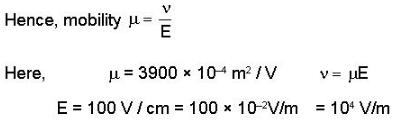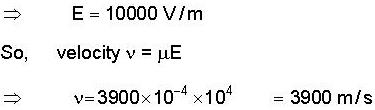QUESTION: 30

A CE amplifier has voltage gain of 50, an input impedance of 1000Ω and an output impedance of 200Ω. The power gain of the amplifier will be :

Solution:

The power gain is defined as ratio of output power to the input power and is given power gain = Voltage gain x current gain =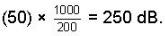*Multiple options can be correct
QUESTION: 31

An amplifier has an open loop gain of 100, an input impedance of 1kΩ & an output impedance of 100 Ω. A feedback network with a feedback factor of 0.99 is connected in a voltage series feedback mode. Choose the correct statement

Solution: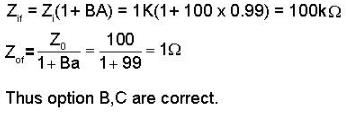*Multiple options can be correct
QUESTION: 32

In a transistor circuit αdc 0.98 and collector to base leakage current (IEBO) = 4μA and the base current is 50μ​A. Choose the correct statement

Solution: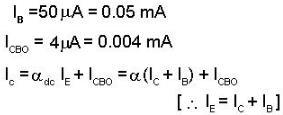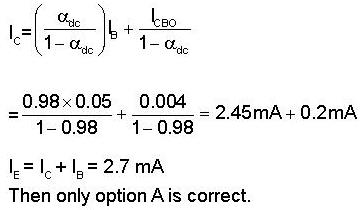*Multiple options can be correct
QUESTION: 33

A voltage 200(100t) is applied to a half wave rectifier with a load Resistance of 5kΩ. Rectifier may be represented by an ideal diode in series with a resistance of 1 kΩ. Which of the following statement is/are correct?

Solution: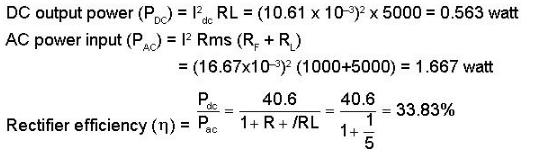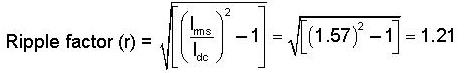*Multiple options can be correct
QUESTION: 34

Which of the curve does not represent the nature of the curve between charge density (ρ) &  distance (r) near the depletion region of p-n junction diode? (where ρ is taken along the y-axis & 'r' is taken along the x-axis)

Solution:

Across p-type & n-type there will be lack of majority charge carriers & excess of minority charge carriers decreases as we move away from junction.

*Multiple options can be correct
QUESTION: 35

Current I1 & I2 flow when large forward voltage V1 & V2 are applied to a semiconductor diode. If C1 = 2V2, then which of the following statement is incorrect expression for the value of reverse saturation current:

Solution: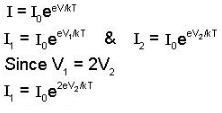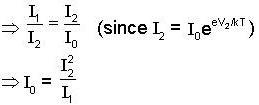*Multiple options can be correct
QUESTION: 36

The temperature of a piece of copper & piece of germanium are cooled from room temperature to 80K. Then which of the following statement are correct?

Solution:

When a piece of Cu & Ge are cooled from room temperature to 80k, then :-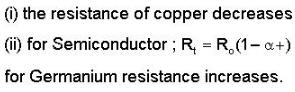*Multiple options can be correct
QUESTION: 37

The Conductivity of a p-type Germanium at 300 k is 100(ohm-cm)-1. The intrinsic concentration of germanium at 300 k is 2.5 x 1013/cm3 & its mobility is given as 1800 cm2/v-s & atoms/cm3 = 4.4 x 1022. Which of the following statement is/are correct, If a voltage source of 0.010V is applied across the semiconductor & the dimension of the p-type germanium piece is (1 x 1) m:-

Solution: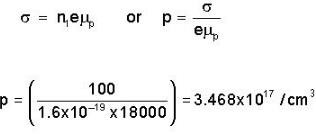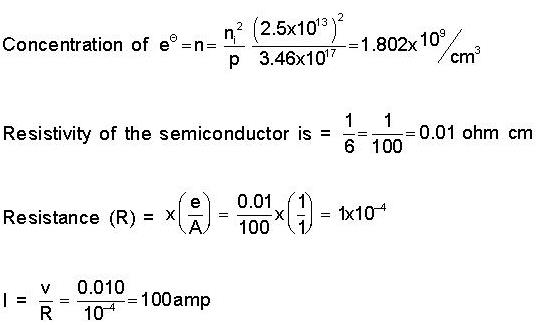*Multiple options can be correct
QUESTION: 38

On the basis of dimensional equation, the maximum number of unknown that can be found, is

Solution:

The maximum number of the unknowns that can be found, on the basis of the dimensional equation of a particular physical quantity are three.

*Multiple options can be correct
QUESTION: 39

The band gap at 300 K of  diamond cubic crystals of C, Si, Ge and Sn is 5.5, 1.2, 0.65 and 0.1 eV respectively. Identify the crystal which will have the highest electrical conductivity at 300K?

Solution:

When energy gap is higher, than conductivity decreases and when energy gap is low, then electrical conductivity is higher (increases).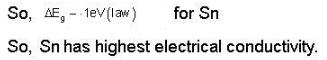*Multiple options can be correct
QUESTION: 40

A bipolar junction transistor used in common collector configuration has

Solution:

A bipolar junction transistor in cc configuration has high input impedance and low output impedance.

*Answer can only contain numeric values
QUESTION: 41

If an amplifier with open loop gain of -1000 and feedback gain of β = -0.1 had a gain change of 20% due to temperature, calculate the change in gain of the feedback amplifier (in %)?

Solution: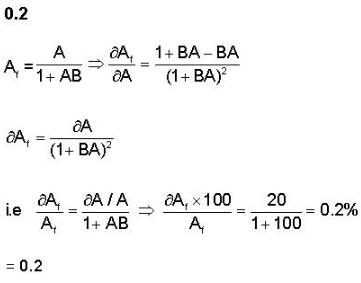*Answer can only contain numeric values
QUESTION: 42

An op-amp has an open loop gain of 105 and an open-loop upper cut-off frequency of 10 KHZ. If this op-amp is connected as an amplifier with a closed-loop gain of 100, calculate the new upper cut off frequency(in Hz)?

Solution: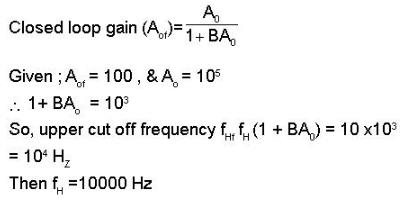*Answer can only contain numeric values
QUESTION: 43

A non-inverting amplifier with gain A = 50,000 Input voltage (V1) = 0.5V average d.c with an a.c sine wave component of 0.3 V peak to peak. Calculate the closed loop gain if R1 = 100 kΩ & Rf = 900 kΩ?

Solution: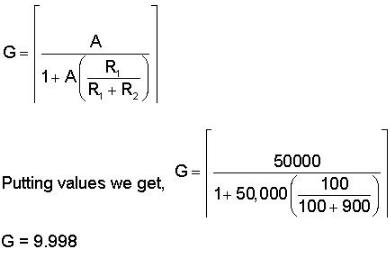*Answer can only contain numeric values
QUESTION: 44

The current flowing in a certain p-n junction at room temperature is 2 x 10-7 A, when a large reverse bias voltage is being applied. Calculate the current (in μA) when a forward voltage of 0.1 is applied across the junction?

Solution: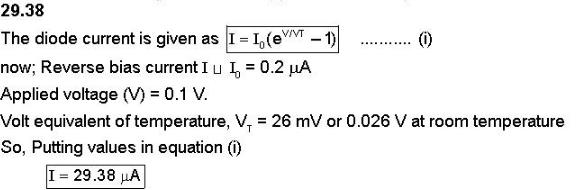*Answer can only contain numeric values
QUESTION: 45

A semiconductor has an electron concentratiion of 0.45 x 1012m-3 & a hole concentration of 5.0 x 1020 m-3. Calculate its conductivity (in SI). (Given electron mobility = 0.135 m2V-1S-1 hole mobility = 0.048m2V-1S-1.)

Solution: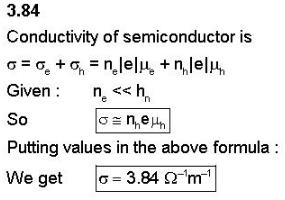*Answer can only contain numeric values
QUESTION: 46

Calculate the magnitude of output voltage "Vo" for the following op-amp circuit with values as given in figure:-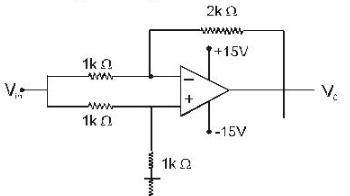Solution: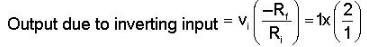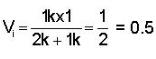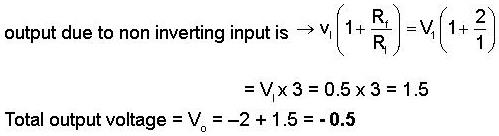*Answer can only contain numeric values
QUESTION: 47

A n-type silicon rod of length 0.7cm has a cross-sectional area of 0.1 cm2. A DC bias, voltage of 35V across on the rod results in a 5.6 A DC current. Under this DC bias an electric pulse applied at one end takes 10ms to traverse the length of Si rod. Calculate mobility of charge carriers.

Solution: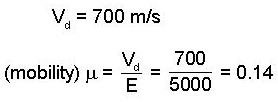*Answer can only contain numeric values
QUESTION: 48

Find the mobility  (in cm2/volt-sec) of e- in copper assuming that each atom contributes one free e- for conduction.
Resistivity of copper is 2.7 x 10-6 ohm-cm.
Atomic weight of copper is 63.54.
Density of copper = 8.96 gm/cm3
Avogadro's number = 6.025 x 1023
Electronic charge = 1.6 x 10-19 coulomb.

Solution: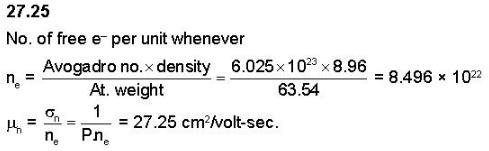*Answer can only contain numeric values
QUESTION: 49

Xener crystallizes in fcc lattice & the edge of the unit cell is 610 pm. Calculate the radius of the Xener atom? (in pm)

Solution: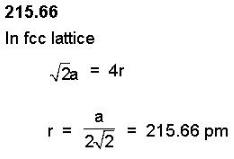*Answer can only contain numeric values
QUESTION: 50

What is the value of the diode current in the circuit using germanium diode, shown below?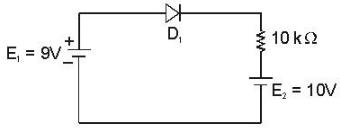Solution:

Diode will be in conducting state, when forward biased voltage is applied to it and in non-conducting state, when reverse biased voltage is applied to it. Now the two batteries may be replaced by a single battery of 1 V. Which will make the diode reverse biased and diode goes to non-conducting state and hence, current is zero: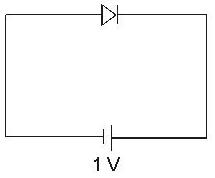*Answer can only contain numeric values
QUESTION: 51

Calculate the saturation collector current (IC(sat)) in following circuit given in figure:-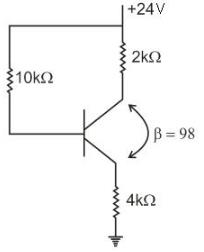Solution: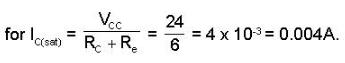*Answer can only contain numeric values
QUESTION: 52

In the circuit given below calculate the value of VCE, if the transistor is working in cut off region.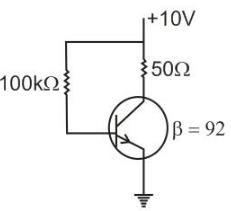Solution: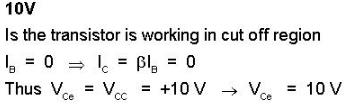*Answer can only contain numeric values
QUESTION: 53

Calculate the output (Vo) of the following opamp circuit:-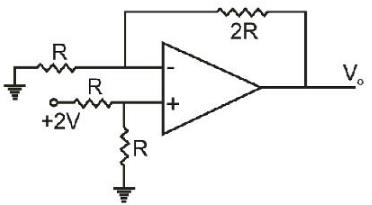Solution: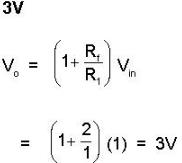*Answer can only contain numeric values
QUESTION: 54

Calculate the d.c. voltage VCE for the circuit given below (in V): (Given : Vbe = 0.7 & β = 50.)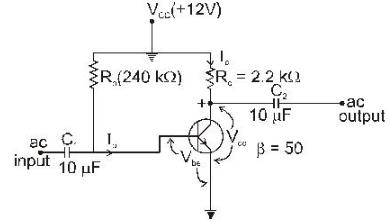Solution: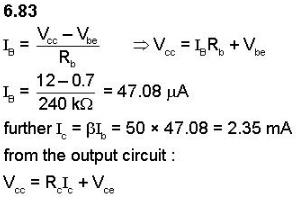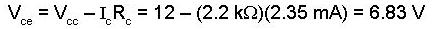*Answer can only contain numeric values
QUESTION: 55

Calculate the potential at point 'y' in the following op-amp circuit.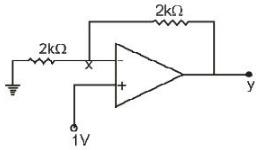Solution: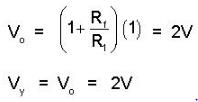*Answer can only contain numeric values
QUESTION: 56

In the transistor circuit given below ; the base current if 35μA, what is the value of base resistor "Rb"? (Given :- RL = 10KΩ)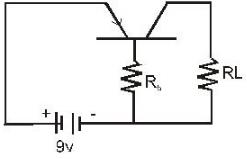Solution: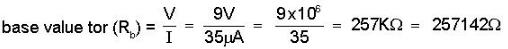*Answer can only contain numeric values
QUESTION: 57

In a transistor the change in base current from 100μA to 125μA causes a change in collector current from 5mA to 7.5 mA keeping collector to emitter voltage Constant at 10V. What is the current gain of the transistor?

Solution: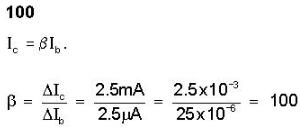*Answer can only contain numeric values
QUESTION: 58

A Zener diode has breakdown voltage of 9.1 V with maximum power dissipation of 364m W. What is the maximum current (in amperes) that the diode can with stand if the total output being supplied to the circuit is 910 mW.

Solution: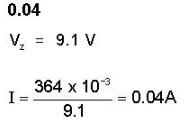*Answer can only contain numeric values
QUESTION: 59

An npn transistor circuit shown in the figure has α = 0.985 VBE = 0.3 volt, R2 = 20 kΩ & Re = 20kΩ, Q-point (2mA, 5V).If VCC = 20 V, then calculate the value of 'R1'? (in kΩ)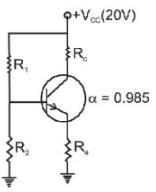Solution:

Using the thevenin equivalent:-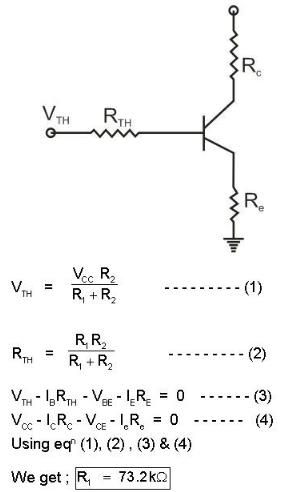*Answer can only contain numeric values
QUESTION: 60

A solid with FCC structure has lattice constant 4.0 A0. Each lattice point has an atom of mass 4.0 x 10-26 kg. Calculate the mass density of the solid?

Solution: